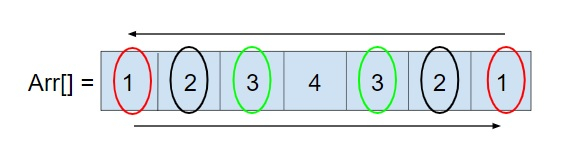# Program to check if an Array is Palindrome or not using STL in C++

Given an array arr[n] of n integers, the task is to find whether array is a palindrome or not. We have to do the stated task using STL in C++.

In C++ there is a feature of STL(Standard Template Library), it is a set of C++ template classes which are used to provide the data structures and several functions such as stacks, queues, lists, etc. To use these one must have knowledge of template classes.

Palindrome is a sequence which is read the same from the front or rear of the sequence. Some simple examples of a palindrome are − MADAM, RACECAR, etc. An array would be a palindrome like in the given example below −So, the task is simple to find out whether the array is a palindrome or not using STL in C++ and print “its a palindrome” if it is a palindrome else “its not a palindrome” if it is not a palindrome.

Input

arr[] = {1, 2, 3, 5, 3, 2, 1}

Output

its a palindrome

Input

arr[] = {1, 2, 3, 4, 5}

Output

its not a palindrome

## Approach used below is as follows to solve the problem

• Set flag = 0 by default in the starting.

• Loop the array i from 0 till size n/2

• For every i check if arr[i]! = arr[n-i-1] then set the flag = 1 and break

• After the loop has ended, If flag is 1 the print “its a palindrome” else print “its not a palindrome”.

## Algorithm

Start 1→ declare function to check if an array is palindrome or not
void check_palindrome(int arr[], int size)
declare int flag = 0
Declare int arr_2[size]
Call memcpy(arr_2, arr, size * sizeof(int))
Call reverse(arr, arr + size)
Loop For int i = 0 and i < size and i++
IF (arr[i] != arr_2[i])
Set flag = 1
Break
End
IF (flag == 0)
Print its a palindrome
End
Else
Print its not a palindrome
End
Step 2→ In main()
Declare int arr[] = { 2,3,4,3,2 }
Declare int size = sizeof(arr) / sizeof(arr)
Call check_palindrome(arr, size)

## Example

Live Demo

#include <bits/stdc++.h>
using namespace std;
void check_palindrome(int arr[], int size){
int flag = 0;
int arr_2[size];
memcpy(arr_2, arr, size * sizeof(int));
reverse(arr, arr + size);
for (int i = 0; i < size; i++)
if (arr[i] != arr_2[i]){
flag = 1;
break;
}
if (flag == 0)
cout << "its a palindrome\n";
else
cout << "its not a palindrome\n";
}
int main(){
int arr[] = { 2,3,4,3,2 };
int size = sizeof(arr) / sizeof(arr);
check_palindrome(arr, size);
return 0;
}

## Output

If run the above code it will generate the following output −

its a palindrome